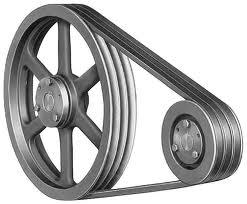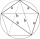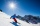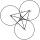# V-belt

Calculate the length of the belt on pulleys with diameters of 105 mm and 393 mm at shaft distance 697 mm.

Result

l =  2.2 m

#### Solution:

$\ \\ r_1 = d_1/2 = 52.5 \ mm \ \\ r_2 = d_2/2 = 196.5 \ mm \ \\ \Delta r = r_2-r_1 = 144 \ mm \ \\ \alpha = arctg(\dfrac{\Delta r} {l}) = arctg(\dfrac{ 144 } { 697 }) = 11.67^\circ = 0.203733309106 \ \\ dr = \sqrt{ l^2-(\Delta r)^2 } = \sqrt{ 697 ^ 2-( 144 )^2 } = 681.96 \ mm \ \\ l_1 = r_1\cdot (\pi-2\cdot \alpha) = 143.54 \ mm \ \\ l_2 = r_2\cdot (\pi+2\cdot \alpha) = 697.39 \ mm \ \\ l = l_1+l_2 + 2 \cdot dr = \dfrac{ 143.54+697.39+2 \cdot 681.96 }{1000} = 2.2 \ \text{m} \ \\$Our examples were largely sent or created by pupils and students themselves. Therefore, we would be pleased if you could send us any errors you found, spelling mistakes, or rephasing the example. Thank you!

Leave us a comment of this math problem and its solution (i.e. if it is still somewhat unclear...):Be the first to comment!Tips to related online calculators
Pythagorean theorem is the base for the right triangle calculator.

## Next similar math problems:

1. Triangle - is RT?Triangle has a circumference of 90 cm. Side b is 1 cm longer than c, side c is 31 cm longer than side a. Calculate the length of sides and determine whether triangle is a right triangle.
2. RT - inscribed circleIn a rectangular triangle has sides lengths> a = 30cm, b = 12.5cm. The right angle is at the vertex C. Calculate the radius of the inscribed circle.
3. Perimeter of triangleIn triangle ABC angle A is 60° angle B is 90° side size c is 15 cm. Calculate the triangle circumference.
4. Five-gonCalculate the side a, the circumference and the area of the regular 5-angle if Rop = 6cm.
5. PyramidPyramid has a base a = 5cm and height in v = 8 cm. a) calculate angle between plane ABV and base plane b) calculate angle between opposite side edges.
6. ReflectorCircular reflector throws light cone with a vertex angle 49° and is on 33 m height tower. The axis of the light beam has with the axis of the tower angle 30°. What is the maximum length of the illuminated horizontal plane?
7. KLM triangleFind the length of the sides of the triangle KLM if m = 5cm height to m = 4.5 cm and size MKL angle is 70 degrees.On a straight stretch of road is marked 12 percent drop. What angle makes the direction of the road with the horizontal plane?
9. MapleMaple peak is visible from a distance 3 m from the trunk from a height of 1.8 m at angle 62°. Determine the height of the maple.
10. Black diamond runTaleah is skiing down a black diamond run. She begins skiing at the top of a ski trail whose elevation is about 8625 feet. The ski run ends toward the base of the mountain at 3800 feet. The horizontal distance between these two points is about 4775 feet.
11. Reference angleFind the reference angle of each angle:
12. If theIf the tangent of an angle of a right angled triangle is 0.8. Then its longest side is. .. .
13. Theorem proveWe want to prove the sentence: If the natural number n is divisible by six, then n is divisible by three. From what assumption we started?
14. CirclesThree circles of radius 95 cm 78 cm and 64 cm is mutually tangent. What is the perimeter of the triangle whose vertices are centers of the circles?
15. TreeHow tall is the tree that observed in the visual angle of 52°? If I stand 5 m from the tree and eyes are two meters above the ground.
16. Vertices of RTShow that the points P1 (5,0), P2 (2,1) & P3 (4,7) are the vertices of a right triangle.
17. High wallI have a wall 2m high. I need a 15 degree angle (upward) to second wall 4 meters away. How high must the second wall?